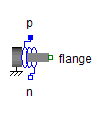Voltage Center Tap 2 m Pulse - MapleSim Help

Voltage Center Tap2m Pulse

Control of 2m pulse center tap rectifierDescription General information about controllers is summarized in Control modelica://Modelica.Electrical.PowerConverters.ACDC.Control .Variables

 Name Value Units Description Modelica ID $\mathrm{twomPulse}$ twomPulse $\mathrm{\delta }$ Delta connection delta $\mathrm{voltageSensor}$ Voltage sensor voltageSensor $\mathrm{gain}$ gainConnections

 Name Description Modelica ID $\mathrm{firingAngle}$ Firing angle (rad) firingAngle $\mathrm{ac}$ ac ${\mathrm{fire}}_{p}$ fire_p ${\mathrm{fire}}_{n}$ fire_nParametersGeneral Parameters

 Name Default Units Description Modelica ID $m$ $3$ Number of phases m f $50$ $\mathrm{Hz}$ Frequency f Use Constant Firing Angle $\mathrm{true}$ True (checked) means use constant firing angle instead of signal input useConstantFiringAngle Constant Firing Angle $0$ $\mathrm{rad}$ Firing angle constantFiringAngle Firing Angle Max. $\mathrm{\pi }$ $\mathrm{rad}$ Maximum firing angle firingAngleMaxFilter Parameters

 Name Default Units Description Modelica ID Use Filter $\mathrm{true}$ True (checked) means enable use of filter useFilter ${f}_{\mathrm{cut}}$ $2f$ $\mathrm{Hz}$ Cut off frequency of filter fCut ${v}_{\mathrm{start}}$ $\mathrm{zeros}\left(m\right)$ $V$ Start voltage of filter output vStartModelica Standard Library The component described in this topic is from the Modelica Standard Library. To view the original documentation, which includes author and copyright information, click here.